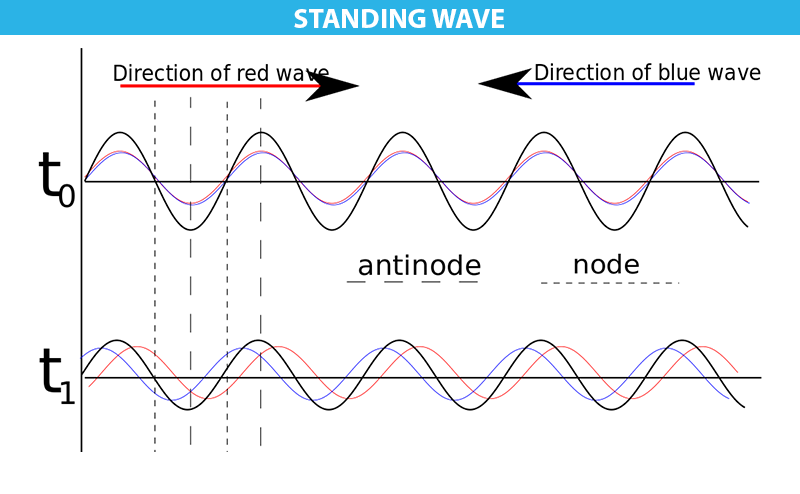# Standing Waves and Normal Modes

Let us consider a system in which a stretched string is bounded at both ends, and we send a continuous sinusoidal wave of a certain frequency towards the positive x-direction. When the wave reaches the right fixed end, it reflects back and travels to the left end. In this process, the left going wave overlaps the wave travelling to the right. The same cycle proceeds, as the left going wave, reflects the left end and starts to travel right, it overlaps the left-going wave. This results in the generation of many overlapping waves which interfere with each other. Both longitudinal waves (e.g sound) and transverse waves (e.g. water) can form standing waves.

### Equation of Standing Wave:

• Let us consider, at any point u and time t, there are two waves, one moving to the left and the other moving to the right. The wave travelling in the positive direction of the x-axis is given as,

y1(u, t) = a sin (ku – ωt),

and that moving in the negative direction of the x-axis is given as,

y2(u, t) = a sin (ku + ωt),

As per the principle of superposition, the combined wave is represented as,

y (u, t) = y1(u, t) + y2(u, t)

= a sin (ku – ωt) + a sin (ku + ωt)

= (2a sin ku) cos ωt• Here, the term called (2a sin ku) provides the amplitude of the oscillation of all the elements of the wave at a position ‘u’ and we also notice that the wave which is represented by this type of equation need not be described as a travelling wave, or as a waveform that does not move to either side. The expression represents the standing wave, wherein the point of minimum, maximum, and the null remains at only one position throughout its propagation.
• The amplitude is said to be zero for all the values of ku that give sin ku = 0. Those values which are given by the ku = nπ, for the values n = 0, 1, 2, 3, …
• Substituting the values k = 2π/λ in expression for the amplitude, we get
$$\begin{array}{l}u=\frac{n\lambda}{2}\end{array}$$
, for the values of n = 0, 1, 2, 3, …

• The positions of the zero amplitudes are called nodes. The distance of 2λ or say half a wavelength will separate the two consecutive nodes.
• The amplitude is said to have a maximum value of 2a, that occurs for all the values of ku that give ⎢sin ku ⎢= 1.
• The values are ku = (n + ½) π for all the values of n = 0, 1, 2, 3, … Hence, by substituting the value for k = 2π/λ in given expression, we get the value for
$$\begin{array}{l}u=\frac{(n+\frac{1}{2})\lambda }{2}\end{array}$$
for the values of n = 0, 1, 2, 3, … as that of the positions for maximum amplitude. These are called as the Anti-nodes. These antinodes are said to be separated by λ/2 and located about half a way between the pairs of nodes. Let’s consider a string with length L whose ends are fixed. The two ends of this string are called nodes.

### Nodes And Antinodes:

• A node is a point along with a standing wave where the wave has minimum amplitude.

• The opposite of a node is an antinode, a point where the amplitude of the standing wave is a maximum. These occur midway between the nodes.

### Normal Mode:

A mass on a spring has one natural frequency at which it freely oscillates up and down. A stretched string with fixed ends can oscillate up and down with a whole spectrum of frequencies and patterns of vibration. These special “Modes of Vibration” of a string are called standing waves or normal modes.

Stay tuned with BYJU’S to explore interesting topics on longitudinal waves, types of waves and many more. Join us and fall in love with learning.

## The video about standing wave on a string## Frequently Asked Questions – FAQs

Q1

### What is a wave?

A wave is a moving, dynamic disturbance of one or multiple quantities. A wave can be periodic in which such quantities oscillate continuously about an equilibrium (stable) value to some arbitrary frequency.
Q2

### What are the main types of waves?

Transverse and longitudinal waves are the main types of waves.
Q3

### What is a standing wave?

A standing wave is a superposition of two distinct waves which creates a wave that changes in amplitude but does not propagate.
Q4

### What is a node?

A node is a point along with a standing wave where the wave possesses the minimum amplitude.
Q5

### What is an antinode?

An antinode is the opposite of a node, a point where the amplitude of the standing wave is a maximum. These exist midway between the nodes.
Test your knowledge on Standing Wave Normal Mode